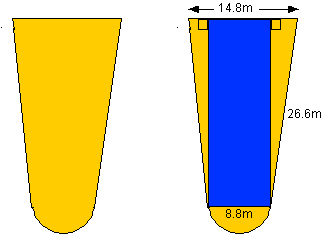PRINT THIS ANSWER SHEET TO FILL OUT AND TURN IN.

NAME_________________________________ CLASS____________________ DATE____________

### Beginner's Guide to Aerodynamics

Drag Equation - Level 2 - Worksheet

In Drag Equation Level 1, our pilot flew his first aircraft of the day. If you have not already done so, you may want to go to this activity first to prepare you for the second flight.

1. Since the type of aircraft has changed so has it's wing area. Once again, in order to work with our drag equation, we first need to find it's wing area. NOTE: Find the areas of each common figure and obtain their sum. You may assume the 2 right triangles are congruent.2. The drag coefficient for our second aircraft was calculated at .365. When the pilot reached the desired cruise speed of 400 mph, the air density was .00052 slugs/cu. ft. What was the aircraft's thrust? NOTE: From the units table in Drag Equation Level 1, you must first convert the area to the proper units. (1 inch = 2.54cm.)

Step 1: Convert area to proper units.

Step 2: Determine the thrust of the aircraft.

3. The thrust of the first aircraft the pilot flew (Drag Equation Level 1) under these same conditions is 150,000 pounds. If the 2 aircrafts weigh the same, which plane is more efficient?